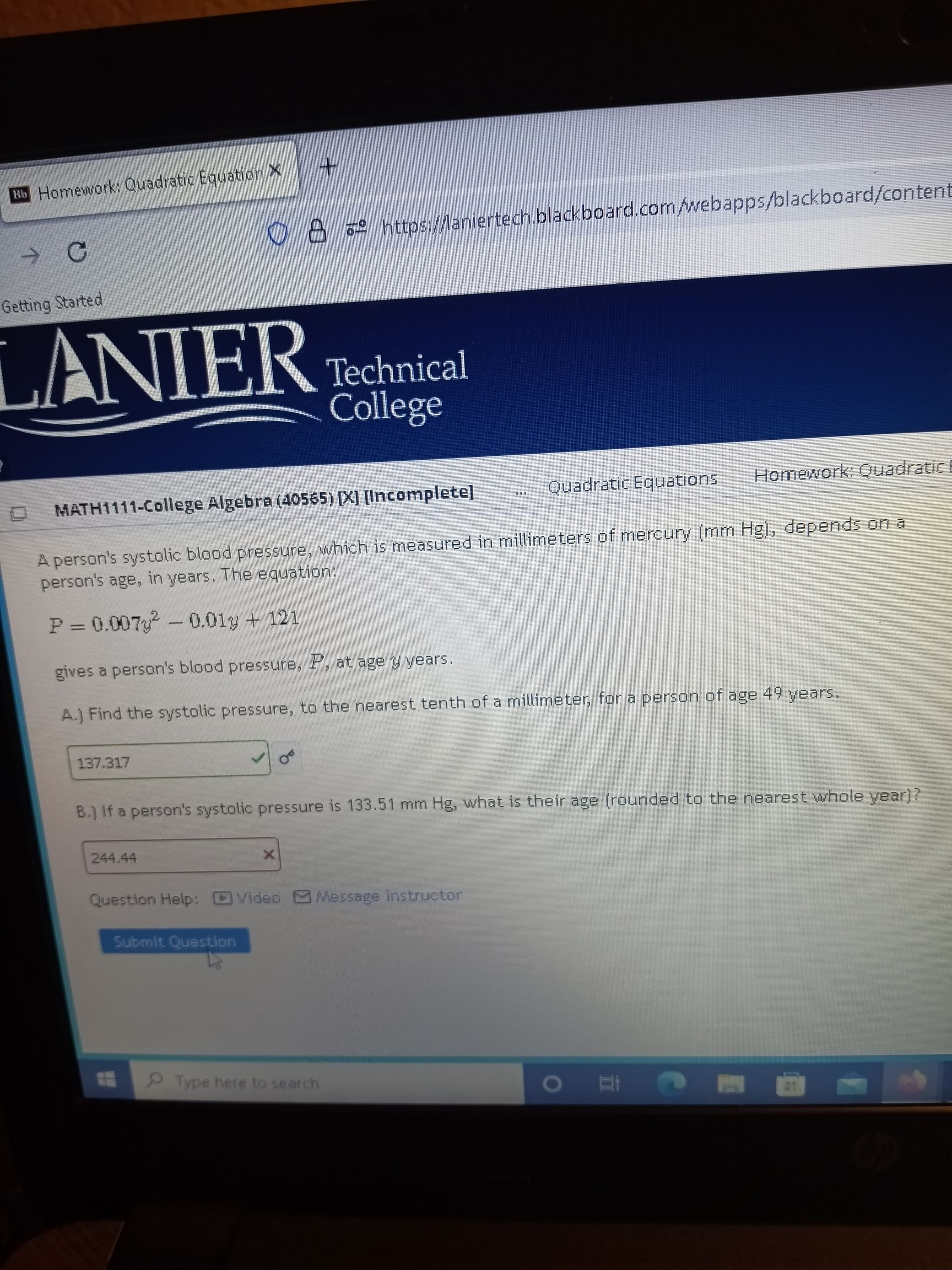### Still have math questions?

Algebra
QuestionA person's systolic blood pressure, which is measured in millimeters of mercuny (mm Hgi, deperids an a person's age, in years. The equation: $$P = 0.007 y ^ { 2 } - 0.01 y + 121$$

gives a person's blood pressure, P, at age $$y$$ years.

If a person's systolic pressure is $$133.51 mm Hg$$ , what is their age (rounded to the nearest whole year)?